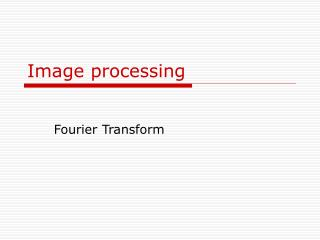DownloadDownload PresentationImage processing

# Image processing

Download Presentation## Image processing

- - - - - - - - - - - - - - - - - - - - - - - - - - - E N D - - - - - - - - - - - - - - - - - - - - - - - - - - -
##### Presentation Transcript

1. Image processing Fourier Transform

2. Discrete Fourier Transform

3. Generate signals t = 0:0.001:0.6; x = sin(2*pi*50*t)+sin(2*pi*120*t); figure, plot(x(1:50)) title('original Signal’) xlabel('time (seconds)') • Add a random noise y = x + 2*randn(size(t)); plot(y(1:50)) title('Signal Corrupted with Zero-Mean Random Noise') xlabel('time (seconds)')

4. Obtain FFT of original and noisy signals X= fft(x,512); Y = fft(y,512);

5. Obtain the power spectrum and plot • The power spectrum, a measurement of the power at various frequencies, is Pxx= X.* conj(X) / 512; Pyy = Y.* conj(Y) / 512; f = 1000*(0:256)/512; figure,plot(f,Pyy(1:257)) title('Frequency content of y') xlabel('frequency (Hz)'); figure,plot(f,Pxx(1:257)) title('Frequency content of x') xlabel('frequency (Hz)')

6. DFT of image C= imread('pout.tif'); y=fft2(C); mesh(abs(y)); The Fourier transform indicates concentration around low frequencies This is due to the slow variations of the intensities (low contrast).

7. Template matching with cross correlation

8. Application • Locate occurrences of the letter "a" in an image containing text. • This is called template matching: Invariant to rotation and shift if performed in Fourier domain • Correlation can be computed by first rotating the image of "a" by 180 and then using the FFT-based convolution technique. • Convolution is equivalent to correlation if you rotate the convolution kernel by 180

9. Obtain image of letter a bw = imread('text.tif'); a=bw(59:71,81:91); %Extract one of the letters "a" from the image knowing the position imshow(bw); figure, imshow(a);

10. Find the max correlation point C = real(ifft2(fft2(bw) .* fft2(rot90(a,2),256,256))); % rotate the image of “a” by 180 (2 times by 90) and pad to fit 256 by 256. figure, imshow(C,[]) max(C(:)) thresh = 45; figure, imshow(C > thresh)